## RENT A THINKER Home My Page Chat Tik-Tok 6        Simulation of Implant of Higher Functions in Randomly Connected Artificial Neural Network.

6.1       ABSTRACT
6.2       INTRODUCTION

6.3       STOCHASTIC TYPE MULTI- LAYER ARTIFICIAL NEURAL NETWORK

6.4       Proposed algorithm

6.5       CHOICE OF HIGHER FUNCTIONAL BLOCKS

6.6       Pre-trained MLP (Multi-Layer Perceptrons) block

6.7       Look up table

6.8       Time delay neuron

6.9       Functional neuron

6.10     PRUNING  ALGORITHM

6.11     RESULTS

6.12     CONCLUSION

### 6.1       ABSTRACT

A large randomly connected Feed Forward type Neural Network (FFNN) is designed to solve a class of problems. This network is used to test and simulate the possibility of hybrid of FFNN and other types of computing elements. The higher functions building blocks are simulated as artificial neurons and are implanted in the above network. The composite network is trained and pruned. As a result, it is observed that, the original network adopts the implanted building blocks and the total size of the network reduces considerably. The suitable reverse characteristics of each functional block are designed to implement training using gradient descendent technique. Some of the building blocks are designed using stochastic type logic. Such building block’s output is similar to the output of the biological neuron. This work is carried out in the view of future possibility of bio-electronic (hybrid) systems.

### 6.2       INTRODUCTION

The objective of our work is to demonstrate the possibility of implantation of non-neural computing devices in the simulated randomly connected Artificial Neural Network (ANN). To test the above possibility, a large randomly connected feed forward type host ANN is designed using a general-purpose neural network tool box . Small independent functional building blocks are designed to simulate artificial neurons. Some of the hidden neurons of the host network is chosen at random and is replaced with above functional blocks. The host network is then trained to solve a specified problem using supervisory learning algorithm. The composite network is then pruned  to optimize the size of the network. The resultant network is a reduced size hybrid of neural and other type of building blocks. It is observed that the implanted functional blocks that were most appropriate to the problem, were adopted by the host network.  Such hybrid system allows computer simulation of large ANN architecture with faster response time. There are three main designing aspects of the above type of network as described below -

·        the stochastic type ANN design,

·        choice of higher function building blocks  and

·        pruning algorithm to optimize the network size.

The following section describes the above aspects in detail. The section II of this paper describes the stochastic  learning algorithm developed for VLSI implementation . The algorithm is similar to the Back Propagation algorithm but can use binary neurons at hidden nodes. Such neuron’s outputs is probabilistic in nature and  resembles output of biological neuron. Section III describes the different types of higher functional building blocks. Using these functional blocks, different applications are developed. Some of the results are given in section V. The Section IV describes the pruning algorithm. An iterative pruning algorithm is developed, which optimizes the network size. In many examples like XOR, circle mapping etc., this algorithm optimizes the network size to the theoretical minimum limit. The section V describes the results of different experiments conducted as mentioned in section II, III and IV. Finally, section VI gives conclusion and useful references are listed at the end.

### 6.3       STOCHASTIC TYPE MULTI- LAYER ARTIFICIAL NEURAL NETWORK

We first review briefly the back propagation (BP) technique  that is popularly used for training FFNNs. The input-output characteristic function (activation function) of a neuron is chosen such that it is continuous and its first derivative is bounded between definite limits for large dynamic range of input. Consider a three layer network of units Yi, Yj and Yk which are interconnected through weights wji and wkj such that-

Xj =  åi wji * Yi;

Yj = f(Xj); (second layer)

Xk = åj wkj * Yj;

Yk = f(Xk); (third layer)

Here Y = f(X) represents the neuron activation function of the form  f(X) = 1/(1+e(-X)).

The weights wkj and wji are modified using the BP algorithm as follows-

wkj = wkj + h * Yj * (Yk-dk) * {Yk * (1-Yk)}                                                                      ....(1)

wji = wji + h *Yi *{Yj *(1-Yj)} * Sk [(Yk-dk)*{Yk *(1-Yk)}* wkj]                                      ....(2)

where h is a real constant less than 1.0 and dk is the desired output. Theoretically, a three-layer network is sufficient for mapping any given input-output set. For reasons of efficiency, however, it may be necessary to consider networks with more than 3 layers. It is straightforward to modify the above equations for the applications having more than 3 layers.

All the variables except Yi and dk of equation (1) and (2) are real variables and hence difficult to implement in VLSI for parallel processing of a large number of perceptrons. It has been shown that the choice of perceptron’s characteristic  is not very critical for convergence of error during training. This paper suggests one such choice that reduces the computational requirement drastically without effecting the error convergence property during training.

### 6.4       Proposed algorithm

We will analyze the significance of each factor in expressions (1) and (2) in order to replace them by suitable probability functions-

[Yk-dk] is the departure of output Yk from the desired value dk.

[Yk * (1-Yk)] is the measure of willingness of Kth output neuron to learn. This is measured by its nearness from its average value (0.5). This factor plays an important role in training.

[wkj  ]  is the back propagation signal path’s conductance.

[Yj * (1-Yj)] is similar to the above term {Yk * (1-Yk )} for hidden layer neuron

[Yi] is the excitation potential for ‘wji.

In the proposed system the perceptron’s forward pass transfer characteristic is generated by a threshold detector having a random bias value as shown in  figure-1. This is equivalent to a choice of input-output characteristic function of equation (3).

Y = 1 for (X + r) > 0; and  Y = 0 for (X + r) <= 0 ;                                                            ...(3)

Here r is a random integer inside the dynamic range of Xj. Yj and Yk are calculated using the above equation -(3) in the forward pass. This has two advantages: the value of Yj is binary but is statistically continuous in the dynamic range of learning and the computations of Xk (for the next layer) does not need any multiplication.

In the backward pass, a term of the form Yk * (1-Yk) is desirable. This term increases the sensitivity of wkj near the threshold value of Xk. Equivalently, the network’s willingness to learn or forget is inversely proportional to the distance of Xj from the threshold. Considering this, a simplified reverse characteristic function R(X), as shown in figure-1 and equation (4), is used to replace the terms of the form {Y * (1-Y)} in equations (1) and (2).

R(X) = 0 for abs(X) > r;  and  R(x) = 1 for abs(X) <= r;                                                            ...(4)

Here r is a positive random integer less than N  and the dynamic range of the X for learning is

-N  to  +N . The value of N is dependent on the network configuration. To improve the dynamic range of the X’s and the rate of convergence, one of the following methods is used -

·        Use N as random integer;

·        Use ‘r’ as weighted random number;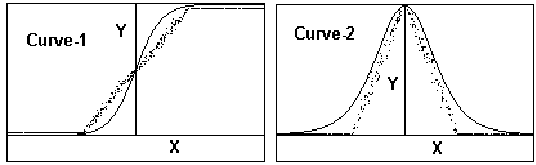Curve-1 (dotted line)        p=N-random(2*N+1)+X if p>0 then z=1 else z=0 Y=(Ss zs)/S  for S samples Curve-1 (solid line) Y = 1 / 1+ e(-X) Curve-2 (dotted line) p=random(N)-abs(X), f p>0 then z=1 else z=0 y=(Ss zs )/S  for S samples Curve-2 (solid line)  Y= e(-X) /(1+ e(-X)) 2

Figure - 1 The forward and the reverse characteristics of the proposed stochastic function   along  with exponential sigmiod function in solid line.

The error convergence curve of a simple 3 layer stochastic type network is shown in figure-2, using XOR input-output function. Figure-3(a) and 3(b) show the output of some of the stochastic type hidden neurons in time domain and the output of a biological neuron respectively. The figure shows the similarity between the stochastic and biological neuron.

### 6.5       CHOICE OF HIGHER FUNCTIONAL BLOCKS

Different types of special type of neuron functions are developed to increase the efficiency of training in a complex network environment. Each of such functional blocks is equivalent to several artificial neurons and associated connections of conventional neural circuit. The functional blocks are implemented using dedicated hardware or micro-processor based system. A general purpose Neural Network Tool Box   is designed to interface different types of functional building blocks with multi-layered FFNN. The Tool Box is a computer simulation program, which supports interactive design of network topology, new activation function and training algorithm. Some of the functions developed using above Tool Box are described below-

### 6.6       Pre-trained MLP (Multi-Layer Perceptrons) block

To reduce the training time, it is convenient to train separate functional blocks of multi-layer FFNN. These functions are generic in nature like image band compression, time series prediction, geometric mapping functions for circle, ellipse, polygon etc.. The pre-trained blocks transfer the BP error like normal FFNN. The elements of these blocks are not reinforced during  training. To simulate above type of pre-trained network block, the Neural Network Tool Box is used. A portion of a large network is first trained using XOR problem. Then the selected weights  are inhibited to reinforce the values during training. The composite network is trained with a function, that uses XOR operation- e.g. (AÅB) OR (cÅD). The figure-  7(a) and 7(b) show the  network, before and after pruning, respectively.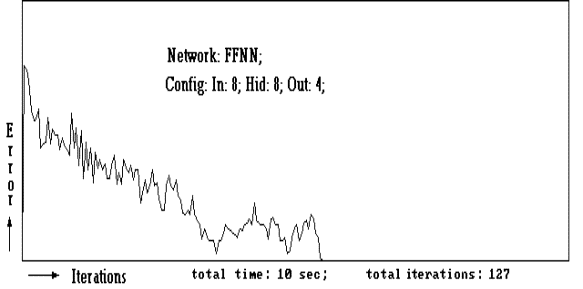Figure - 2  The error convergence curve of a 3 layer stochastic type Feed Forward Neural   Network.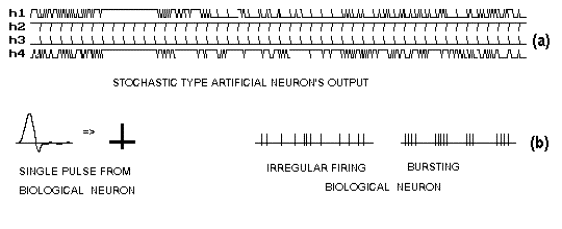Figure- 3  Similarity between  the stochastic and the biological neurons. (a) Output of stochastic  type neurons (see text). The curves   h1, h2, h3  &  h4   are  hidden neuron’s output  during   training   for  fixed  input  pattern.  The  X-axis   represents   iteration  (@ 10  samples per iteration). (b)  Output of biological neurons..

### 6.7       Look up table

For binary input-output operation, boolean functions and look up tables are used, which are integrated with a host network using stochastic learning algorithm. This gives higher flexibility, density and speed. Appropriate reverse characteristics are designed using stochastic type functions.

### 6.8       Time delay neuron

For efficient representation of the time domain signal, time delay neuron is introduced. The time delay neuron retains history of time series input signals in the hidden neurons of  the network. A time delay neuron receives the input from the immediate neighboring neuron, retains the value for ‘d’ period of integer delay and outputs like normal neuron. These neurons allow variable unit of integer time delay as shown in the equation (5).

Yj(t) = Xj(t-dj)                                                                                                 ...(5)

where ‘djis the integer time delay of j th   delay-neuron and ‘t’ is the time.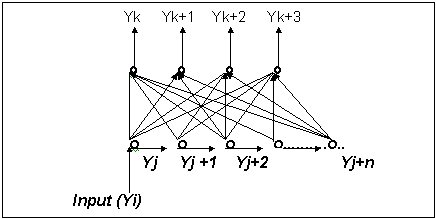Figure - 4  The organization of the time delay neuron.The total time delay  Td  of all the  neurons  in  a  time  delay  network is given by -  Td=Sj dj.   Figure-4 shows the organization of time delay neurons as a functional building block. There is a single input Yi, which is delayed through different delay neurons Yj. The output of delay neurons Yj s are connected to Yks, the output neurons. The above building block retains the time domain information in Yj neurons. Using such building blocks, it is possible to generate functions of the convolution of input signal, standard filters, time series prediction etc.. One of such applications shown in figure-5 is time series prediction. The network of figure-4 is trained to predict a complex time series function.

### 6.9       Functional neuron

Functional neuron is a neuron that is pre-defined function (equation) which may be equivalent to several neurons and interconnections. One of the trigonometric and algebraic functions are  studied are given in equation (6).

Xj = ( åi (W * Yi 2) ) 0.5  ;                                                                                         ...(6)

The use of functional neuron drastically reduces the network size and increases the speed of operation. These types of neurons are appropriate for real time applications for modeling, image prediction, pattern recognition etc. Use of functional neurons also facilitates in building mathematical model from the observed data. This is achieved by studying the  topology and inter-relationship of   functional neurons inside the network. Section-V describes the results of circular boundary mapping using the neuron activation function of equation (6).

The method described above are integrated with a host network. For each method suitable error propagation strategy is developed, to reinforce the components of the host network. In case of the functions, which are not differentiable, the stochastic method as described in section II, is used. The composite network is simplified using pruning algorithm as described in section below.

### 6.10  PRUNING  ALGORITHM

A simple but efficient technique is developed which reduces the size of the network almost to the theoretical limit with minimum number of trials. The method is based on the simple fact that in a trained network, useful connections are stronger and the unwanted connections are weaker. The effect is further enhanced by slowly reducing the strength of all the interconnections with time. The  operation is  analogous  to  forgetting.  When  learning  and  forgetting  reaches equilibrium,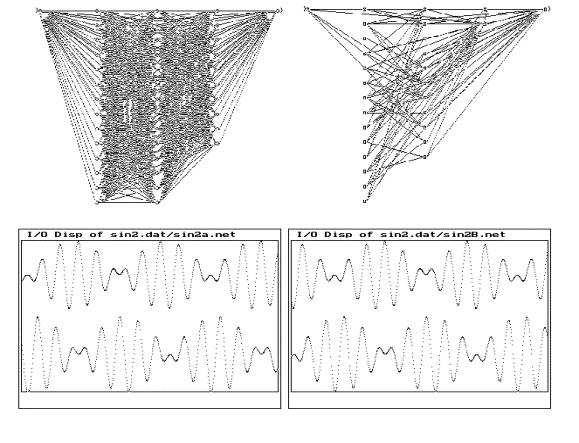Figure - 5 (a)  A fully connected FFNN configuration with a time-delay functional block trained for time series prediction.  (b) The resultant network after training and pruning.  (c) & (d) The input signal (top trace) and the predicted output(bottom trace) of the network (a) & (b).

the optimum configuration connections are stabilized. When equilibrium is reached, the weakest connections are gradually eliminated using successive approximation method, until the network’s output error is less than or equal to a desired error limit. As a result of the reduced connections, some neurons will have no input or will have only single input-output connection. These neurons are eliminated by using appropriate rules and connections are rerouted. The figure-6 shows the Flow-chart of the pruning algorithm. The figure-5, 7, 8 and 9 shows the result of pruning in different applications.

### 6.11  RESULTS

Using the above techniques, several experiments were conducted to test the feasibility of implanting non-neural functional blocks, to a redundantly connected large host FFNNs. In most cases, it was observed that, the host network adopts the implanted functional blocks. The implanted functional blocks participate in solving problems and a large portion of the other network elements becomes redundant. Hence, the network size minimizes considerably after pruning. Some  of  the cases studied are given below-

Experiment - I :  XOR problem

A fully connected multi-layer FFNN is configured using two pre-trained network h1, h2. h5, P  and h3, h4, h6, Q. The network has four inputs a, b, c, d and one  output R,  as  shown  in  the   figure-7(a). The network is trained and pruned as explained in section-IV  using the function -

R = (AÅ B) OR (CÅ D).

After pruning, the networks corresponding to P and Q are automatically isolated from each other. Also the four redundant hidden neurons are eliminated as shown in figure 7(b).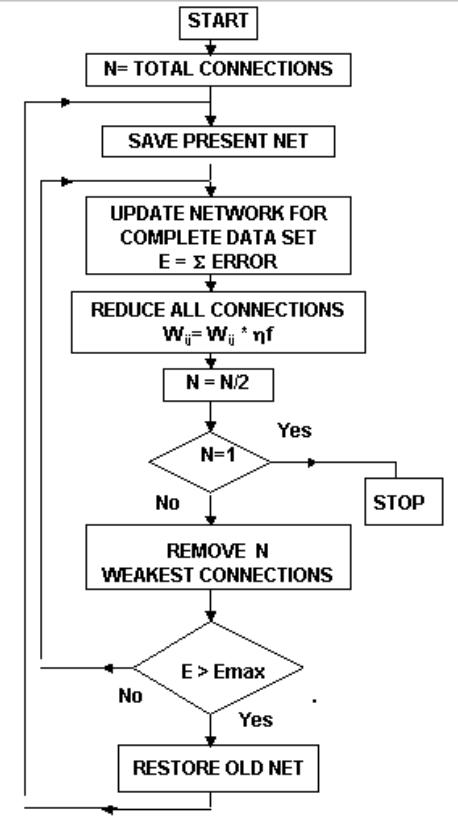Figure-6 Network optimizing algorithm, where Emax is the maximum tolerable error.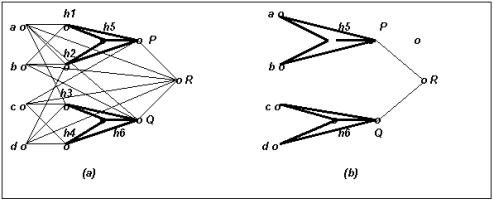Figure-7 (a)  The implantation  of pre-trained XOR Neural  Network- h1, h2, h5, P and h3, h4, h6, Q in a host network. (b) The resultant network  after pruning.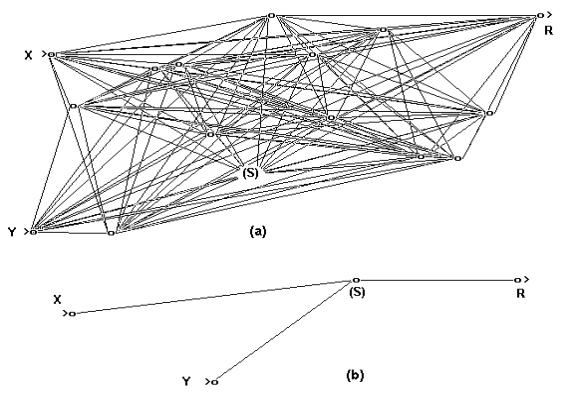Figure-8 (a)In above example let rad=((X-0.5)2+(Y- 0.5)2)0.5 and if (rad > 0.3) then R=0 else R=1. X and Y are random analog inputs between 0 to 1. A special neuron S (See text) is implanted among the hidden neurons in the network. (b) The optimized network, where all the hidden neurons are eliminated except neuron S.

Experiment-II :   Non  linear  Boundary  Mapping  Problem

In this example, a two dimensional image mapping problem is studied. A two layer network as shown in figure-8(a) is configured with two analog inputs, thirteen hidden neurons and a binary output neuron. Twelve hidden neurons are having conventional exponential sigmoid type activation function and only one hidden neuron (S)  is used with   function of equation - (7).

Xj = Si (Wij * Yi2) and  Yj = 1/(1 +e Xj)                                                                  ....(7)

The network is first trained to map a circle and then  is pruned. The pruning result is  shown in  figure-8(b). The resultant network has only one hidden neuron and three connections. The only neuron (S) left in the hidden layer after the pruning operation is the one, which was specially implanted with the activation function of equation-(7). The final network is also the minimum configuration to map circular boundary. The inputs and  output of the network of figure- 8(a) and 8(b) are shown in figure- 9(a), 9(b) and  9(c).

### 6.12  CONCLUSION

It is shown by the simulation that a feed forward type neural network can adopt higher order mathematical building blocks during learning operation. It is shown that such building blocks could use probabilistic pulse frequency modulated signals using stochastic logic. Such implant has similar characteristics as biological neurons. Such experiments are very primitive steps towards direct man machine interface.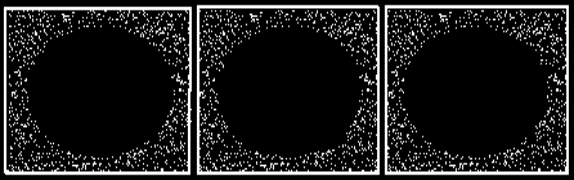(a)                                           (b)                                       (c)

Figure-9  (a) Input training pattern for figure- 8 (a)&(b).  (b)Output response of network of  figure- 8(a).  (c) Output response of network of figure-8(b).

### 6.13  REFERENCES

 Alspector, Allen J., R.B., Hu.V., & Satyanarayana, S., “ Stochastic learning networks and    their implementation “. In D.Z. Anderson (Ed.), Proceedings of the IEEE Conference on Neural  Information Processing Systems - Natural and Synthetic, New York: American Institute of  Physics, 1988, pp. 9-21.

 Baum Eric. B and Haussler David “ What Size Net Gives Valid Generalization ?”.Neural Computation, issue of January, 1989.

 Karnin E. D, “A simple procedure for pruning back-propagation trained neural network,” IEEE  Trains. Neural Networks, vol. 1, June, 1990, pp. 239-244.

 Lippmann R.P., An introduction to computing with Neural Nets. IEEE ASSP Magazine, April 1987, pp. 4-22,

 Mazumdar Himanshu S, “A mlutilayered feed forward neural network suitable for VLSI implementation“, Microprocessors and Microsystems, vol. 19, number 4, May, 1995, pp. 231-234.

 Rawal Leena P and Mazumdar Himanshu S,     “ A    Neural   Network Tool  Box  using   C++“,  Computer Society of India, vol. 19, number 2, August 1995, pp. 15-23.

 Rumelhart D.E., Hinton G.E., and Williams R.J.,” Learning Representations by Back-Propagation Errors”,Nature, Vol.323, No.9, Oct. 1986, pp. 533-536.

6.14  Published

Mazumdar Himanshu S.  and Rawal Leena P., "Simulation  of Implant of higher Functions in Randomly  Connected  Artificial  Neural Network", published  in  the  Abstracts  proceedings,   main  papers  XIVA. The International Conference on Cognitive Systems 1995, Dec. 15th & 16th, New Delhi, Organized by the R & D Center, NIIT Ltd., INDIA.